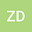loading page

LIMIT CYCLES IN DISCONTINUOUS GENERALIZED LIÉNARD DIFFERENTIAL EQUATIONS
•• Zouhair Diab
Zouhair Diab
University of Tebessa
Author Profile## Abstract

The goal of this paper is to study the number of limit cycles that can bifurcate from the periodic orbits of a linear center perturbed by nonlinear functions inside the class of all generalized Liénard di¤erential equations allowing discontinuities. In particular our results show that for any n 1 there are di¤erential equations of the form x¨+f(x; x_ )x_ +x+sgn(x_ )g(x) = 0, with f and g polynomials of degree n and 1 respectively, having [n=2] + 1 limit cycles, where [] denotes the integer part function.